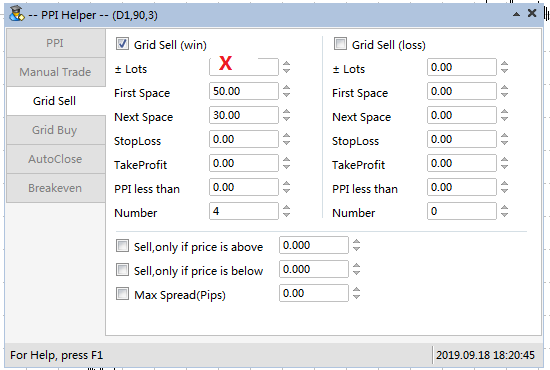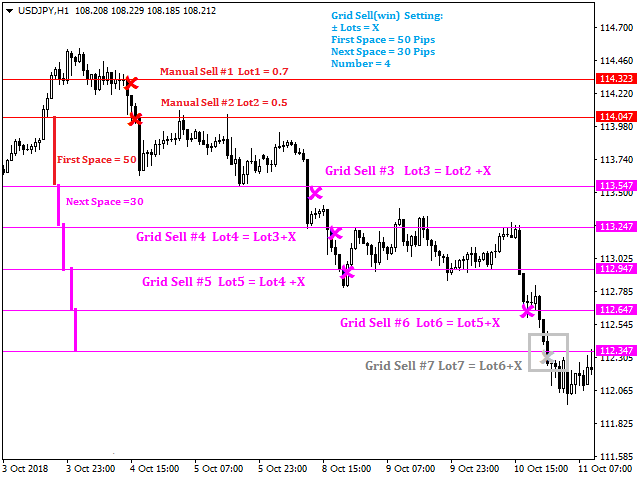Grid Sell(win) ,the rules as below:

• The market is a space below the lowest price of sell orders, EA will add grid sell(win) order.
•  The lots = the last lots + ± Lots
• ± Lots  = 0， the lots will be the same as the last lots
• ± Lots  > 0， the lots will increase
• ± Lots  < 0， the lots will decrease, if the result is negative or zero, EA will not open order.

Suppose the setting as below:

• Must check Grid Sell(win)
• ± Lots X ( it could be zero, positive or negative),
• First Space = 50  it must be positive number
• Next Space = 30  it must be positive number
• StopLoss =0
• TakeProfit = 0
• PPI less than = 0  Only when the real PPI is less than this setting, EA will open order. If do not need the PPI limit,just set it 111.
• Number = 4    the max number of grid sell(win) positions, if it is 0,there is no limit.Initially,we have two sells from manual trade:

Sell#1 Lot1=0.7,

Sell#2 Lot2=0.5;

When the market price is below the lowest price of these two orders.

If the market is a space("First Space") below the lowest price,and there is no grid sell order, EA will open a first grid sell order, Sell #3 Lot3 = Lot2+X

If the market is going down, for every "Next Space", EA will open grid sell oder, Sell#4, Sell#5......1.  if X = 0    -- Lot3 = Lot2+X = Lot2. So Lot3,Lot4,Lot5,and Lot6 are the same as Lot2
2.  if X=0.1   -- Lot3 = Lot2+X = 0.3, Lot4 = Lot3+X = 0.4, Lot5 = Lot4+X = 0.5 ,  Lot6 = Lot5+X = 0.6 ,there are 4 grid sell orders,so EA will not open grid sell#7.
3.  if X= -0.2 -- Lot3 = Lot2+X = 0.5-0.2 = 0.3, Lot4 = Lot3 +X = 0.3 - 0.2 =0.1,  Lot5 = Lot4+X = 0.1 - 0.2 = - 0.1,as the lots is less than zero,EA will not open Sell#5

EA --  PPI Helper:  https://www.mql5.com/en/market/product/42178

Pair Power Index Helper Post https://www.mql5.com/en/blogs/post/729770

Share it with friends: